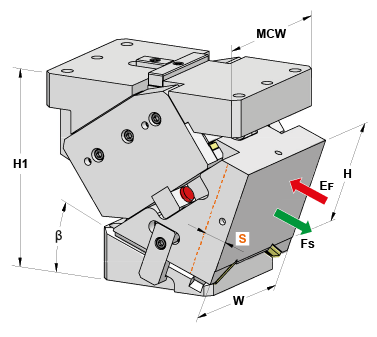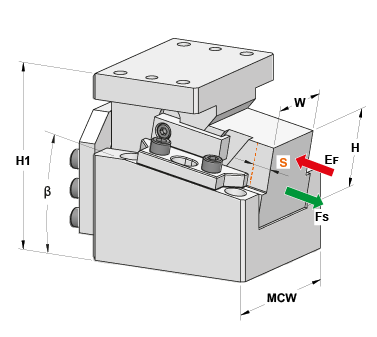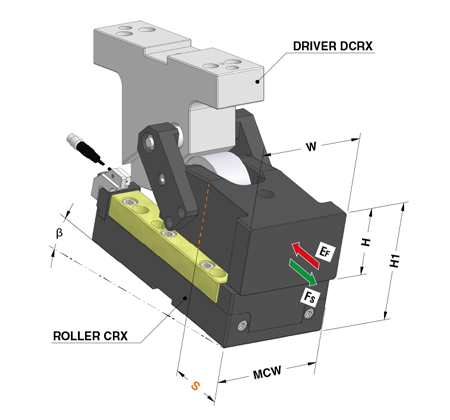## Cam searchCode = OMCR code
β = work angle (°)
S = stroke (mm)
W = slider width (mm)
H = slider height (mm)
H1 = cam unit height (mm)
MCW = maximum cam width (mm)
Fs = max work force shoulder (kN)
Ef (g) = extraction force (kN) with gas spring
Ef (s) = extraction force (kN) with spring
Price = €/unit
WD = delivery time in working days from date receiving order
Weight = kg/unitCode = OMCR code
β = work angle (°)
S = stroke (mm)
W = slider width (mm)
H = slider height (mm)
H1 = cam unit height (mm)
MCW = maximum cam width (mm)
Fs = max work force shoulder (kN)
Ef (g) = extraction force (kN) with gas spring
Ef (s) = extraction force (kN) with spring
Price = €/unit
WD = delivery time in working days from date receiving order
Weight = kg/unitCode = OMCR code
β = work angle (°)
S = stroke (mm)
W = slider width (mm)
H = slider height (mm)
H1 = cam unit height (mm)
MCW = maximum cam width (mm)
Fs = max work force shoulder (kN)
Ef (g) = extraction force (kN) with gas spring
Option K = cam unit without positive return
Option P = cam unit with positive return
Option Driver = cam unit with driver
Option X = cam unit with back position check of the slider
Price = €/unit
WD = delivery time in working days from date receiving order
Weight = kg/unit